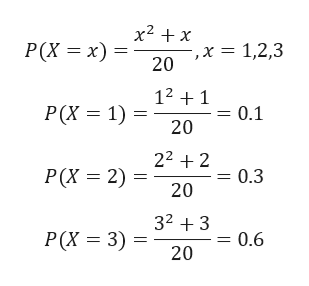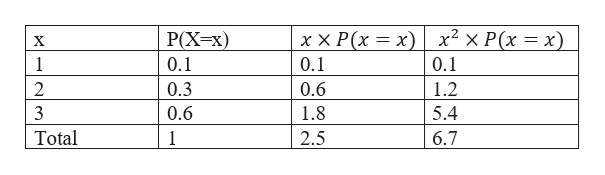a discrete probability distribution is defined by the function P(X = x) = (x^2 + x) / 20 ; x = 1, 2, 3 a) use this information to fill in the second column in the table. The additional columns are for you to use as needed when finishing other parts of this problem. There may be an extra column. xP ( X = x)    1     2     3     total      b) describe how you can determine that the algebraic function does indeed define a discrete probability c) use another column on the table as needed to find the mean, also known as the expected value d) Use another column on the table as needed to find quantities needed to calculate the standard deviation.

Question

a discrete probability distribution is defined by the function

P(X = x) = (x^2 + x) / 20 ; x = 1, 2, 3

a) use this information to fill in the second column in the table. The additional columns are for you to use as needed when finishing other parts of this problem. There may be an extra column.

 x P ( X = x) 1 2 3 total

b) describe how you can determine that the algebraic function does indeed define a discrete probability

c) use another column on the table as needed to find the mean, also known as the expected value

d) Use another column on the table as needed to find quantities needed to calculate the standard deviation.

Step 1

The given discrete probability distribution ishelp_outlineImage Transcriptionclosex2 x P(X x) 20 1,2,3 12 1 Р(X %3D1) = 0.1 20 222 = 0.3 P(X 2) 20 323 Р(X 3 3) 0.6 20 fullscreen
Step 2

a) The calculation is shown in tablehelp_outlineImage TranscriptioncloseхХ Р(x 3 х)| х? Х Р(х %—D х) 0.1 P(Xx) X 1 0.1 0.1 2 0.3 0.6 1.2 3 0.6 1.8 5.4 Total 6.7 1 2.5 fullscreen
Step 3

b) A discrete probability distribution takes values that are countable, basically it takes whole no.

The probability of each discrete random variable is bet...

Want to see the full answer?

See Solution

Want to see this answer and more?

Our solutions are written by experts, many with advanced degrees, and available 24/7

See Solution
Tagged in

Measures